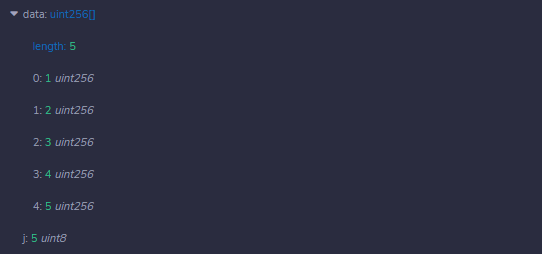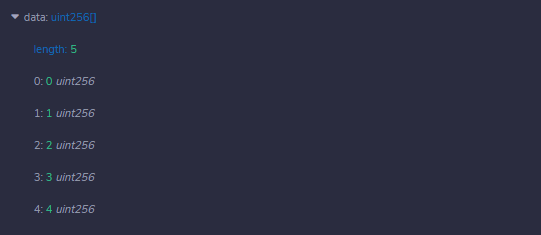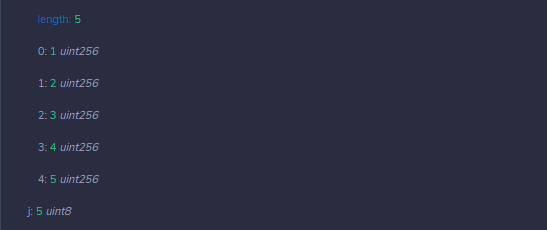# Solidity – While, Do-While, and For Loop

• Last Updated : 11 May, 2022

Loops are used when we have to perform an action over and over again. While writing a contract there may be a situation when we have to do some action repeatedly, In this situation, loops are implemented to reduce the number of lines of the statements. Solidity supports following loops too ease down the programming pressure.

### While Loop

This is the most basic loop in solidity, Its purpose is to execute a statement or block of statements repeatedly as far as the condition is true and once the condition becomes false the loop terminates.

Syntax:

```while (condition) {
statement or block of code to be executed if the condition is True
}
```

Example: In the below example, the contract Types demonstrate the execution of a while loop and how an array can be initialized using the while loop.

## Solidity

 `// Solidity program to  ` `// demonstrate the use ` `// of 'While loop' ` `pragma solidity ^0.5.0;  ` ` `  `// Creating a contract  ` `contract` `Types {  ` `     `  `    ``// Declaring a dynamic array ` `    ``uint[] data;  ` `   `  `    ``// Declaring state variable ` `    ``uint8 j = 0; ` `    `  `    ``// Defining a function to  ` `    ``// demonstrate While loop' ` `    ``function loop( ` `    ``) ``public` `returns(uint[] memory){ ` `    ``while(j < 5) { ` `        ``j++; ` `        ``data.push(j); ` `     ``} ` `      ``return` `data; ` `    ``} ` `}`

Output :### Do-While Loop

This loop is very similar to while loop except that there is a condition check which happens at the end of loop i.e. the loop will always execute at least one time even if the condition is false.

Syntax:

```do
{
block of statements to be executed
} while (condition);
```

Example: In the below example, the contract Types demonstrate the execution of a do-while loop and how an array can be initialized using the do-while loop.

## Solidity

 `// Solidity program to  ` `// demonstrate the use of ` `// 'Do-While loop' ` `pragma solidity ^0.5.0;  ` ` `  `// Creating a contract  ` `contract` `Types {  ` `     `  `    ``// Declaring a dynamic array ` `    ``uint[] data;  ` `   `  `    ``// Declaring state variable ` `    ``uint8 j = 0; ` ` `  `    ``// Defining function to demonstrate  ` `    ``// 'Do-While loop' ` `    ``function loop( ` `    ``) ``public` `returns(uint[] memory){ ` `    ``do``{ ` `        ``j++; ` `        ``data.push(j); ` `     ``}while(j < 5) ; ` `      ``return` `data; ` `    ``} ` `}`

Output :### For Loop

This is the most compact way of looping. It takes three arguments separated by a semi-colon to run. The first one is ‘loop initialization’ where the iterator is initialized with starting value, this statement is executed before the loop starts. Second is ‘test statement’ which checks whether the condition is true or not, if the condition is true the loop executes else terminates. The third one is the ‘iteration statement’ where the iterator is increased or decreased. Below is the syntax of for loop :

Syntax:

```for (initialization; test condition; iteration statement) {
statement or block of code to be executed if the condition is True
}
```

Example: In the below example, the contract Types demonstrate the execution of a while loop and how an array can be initialized using the while loop.

## Solidity

 `// Solidity program to  ` `// demonstrate the use ` `// of 'For loop' ` `pragma solidity ^0.5.0;  ` `  `  `// Creating a contract ` `contract` `Types {  ` `    `  `    ``// Declaring a dynamic array  ` `    ``uint[] data;  ` ` `  `    ``// Defining a function  ` `    ``// to demonstrate 'For loop' ` `    ``function loop( ` `    ``) ``public` `returns(uint[] memory){ ` `    ``for``(uint i=0; i<5; i++){ ` `        ``data.push(i); ` `     ``} ` `      ``return` `data; ` `    ``} ` `}`

Output :My Personal Notes arrow_drop_up
Recommended Articles
Page :